Computational Tricks: Example 1 (page 1 of 2)

When you have a graphing calculator, you can whip out a quick graph of a given polynomial, and get a good idea from the picture of where the x-intercepts (zeroes) are. This is useful, because it can save you from wasting time trying zeroes that aren't reasonable. So what do you do if you don't have a graphing calculator? Do you really have to try all the possible roots that the Rational Roots Test might spit out?

Not necessarily. There are a couple tricks you can use when working with synthetic division and the Rational Roots Test. Here's an example:

• Find all the roots of 2x3 + 7x2 – 16x + 6.

The Rational Roots Test gives the following list of possible zeroes: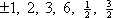For comparison, here is what the graph looks like: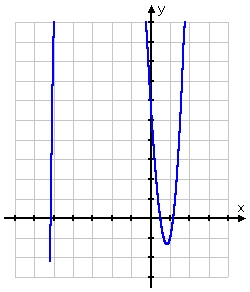As you can see, there are no x-intercepts before x = –6 and no x-intercepts after x = 2. I would guess that the zeroes are a little before x = –5, right at x = 1/2, and a little after x = 1. I would guess that the rational zero will be at x = 1/2, and the other two zeroes will be irrational, generated by the square root in the Quadratic Formula.

But if you don't have a graphing calculator handy, you don't have any of this information. So let's pretend we don't know what the graph looks like, and try x = –6 as our test zero: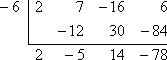As you can see, the remainder is non-zero, so x = –6 is not a solution of 2x3 + 7x2 – 16x + 6 = 0. But I'd like you to notice something else: look at the signs on the numbers in the bottom row. I divided by a negative, and the signs on the bottom row alternated (plus, minus, plus, minus).

The relationship is this: If, when using synthetic division, you divide by a negative and end up with alternating signs on the bottom row, then the test root was too low. (This does not work in reverse! You can sometimes divide by a too-low test root, but not get alternating signs on the bottom row!)

Now let's try a too-high test root. I'll try x = 2 as the test zero: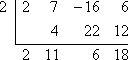As you can see, the remainder is non-zero, so x = 2 is not a solution of 2x3 + 7x2 – 16x + 6 = 0. But I'd like you to notice the bottom row again. I divided by a positive, and the signs on the bottom row are all positive.

The relationship is this: If, when using synthetic division, you divide by a positive and end up with all positive numbers on the bottom row, then the test root is too high. (This does not work in reverse! You can sometimes divide by a too-high test root, but not get all positive numbers on the bottom row!)

In either case, "0" may be counted as positive or negative (though technically it is neither), according to whichever pattern you're trying to match. For instance, if you'd divided by a negative and your bottom row has been "1  –3  2  0  4   –5", then you could have counted the "0" as being negative and the signs would have been alternating, telling you that your test root was too low. In similar manner, if you'd divided by a positive and your bottom row had been "1  3  2  0  4  5", then you could have counted the "0" as being positive and all the signs would have been positive, telling you that your test root was too high. Copyright © Elizabeth Stapel 2002-2011 All Rights Reserved

Warning: These are the only two patterns. If you divide by a negative and get all positives, all negatives, or any pattern other than alternating signs, then the bottom row tells you nothing. You cannot conclude anything about whether or not the test root was too low (or too high). If you divide by a positive and get all negatives, alternating signs, or any pattern other than all positives, then the bottom row tells you nothing. You cannot conclude anything about whether or not the test root was too high (or too low). In practice, this means that generally the bottom row won't tell you much!

Getting back to the problem at hand:

I now know that x = –6 is too low. Wherever my zeroes are, they're above x = –6. So now I'll try x = –2: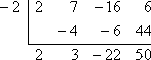The bottom row does not have alternating signs, so I can't tell from this whether or not x = –2 is too low (or too high). (From the picture, we know that it is neither —it is actually between two zeroes—, but we cannot tell this from the synthetic division.)

However, I can conclude something useful from this result. Since the remainder for the first division was –78, this meant that f(–6) = –78, by the Remainder Theorem. This last division says that f(–2) = 50. Since  f(–6) is negative (that is, the graph is below the x-axis at x = –6) and f(–2) is positive (that is, the graph is above the x-axis at x = –2), then the graph must cross the x-axis somewhere between x = –6 and x = –2. That is, there must be a zero of f(x) = 2x3 + 7x2 – 16x + 6 between x = –6 and x = –2. The only candidate (from my list) is x = –3, so I'll try that: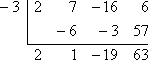Nope; whatever the zero is between x = –6 and x = –2, it isn't rational. However, since f(–3) = 63, which is positive, then I have narrowed down the location of the irrational zero: it will be between x = –6 and x = –3.

I still need to find a zero, so now I'll try x = 1, since I know that x = 2 is too high: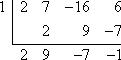While the bottom row is not all positive (so I cannot conclude that x = 1 is too high), I can conclude that a zero must lie between x = 1 and x = 2, because f(1) = –1 is negative and f(2) = 18 is positive. The only rational candidate is x = 3/2, so I'll try that: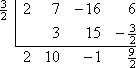Nope; x = 3/2 isn't a zero either. So the zero between x = 1 and x = 2 must be the other irrational root from the Quadratic Formula, the pair to the irrational root between x = –6 and x = –3. The rational root must lie elsewhere.

Since f(–2) = 48 is positive and f(1) = –6 is negative (so there must be a zero between x = –2 and x = 1), one of x = –3/2, x = –1, x = –1/2 and x = 1/2 must be the rational zero.

Note: Descartes' Rule of Signs says that there is one negative root and either two or zero positive roots. Since we have already determined that there is an irrational root between x = –6 and x = –3 (so the negative root has already been partially located), then any rational root must be positive. Therefore, I can cross x = –3/2, x = –1, and x = –1/2 off my list of possible roots.

Okay, now I'll try x = 1/2: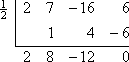Aha! I've finally found my rational root! And dividing out x = 1/2 (that is, dividing out the factor x – 1/2 ) leaves me with a quadratic which I can solve:

2x2 + 8x – 12 = 0

x2 + 4x – 6 = 0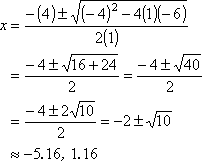Then the zeroes are: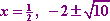These polynomial-solution problems are usually long and annoying like this. You can probably see how looking at a graph can be very useful! But if you don't have access to a graphing calculator, you can (eventually) get the correct answer. I would recommend that you practice, so you can get a "feel" for how these work. That way, you can quickly process these questions, and not waste too much time on your tests.

Now let's do an example without a graph....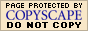WyzAnt Tutoring